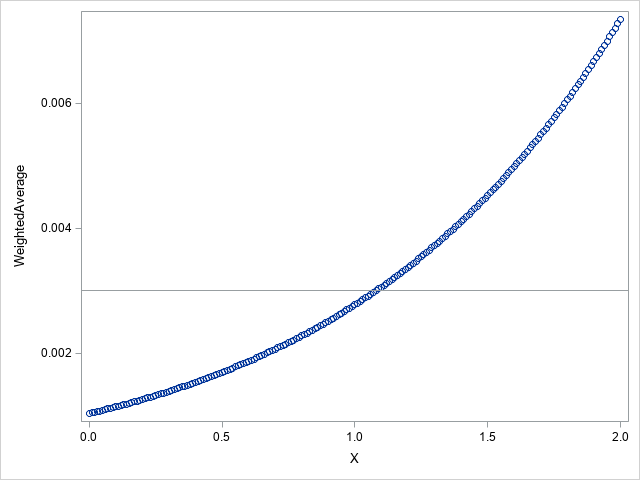## Replicate Solver in excel without PROC OPTMODEL

Hello, I'm a very new SAS user and would like to know if there is any solution to the problem I am facing. I have read through many questions regarding replicating Solver in excel and most of them require SAS/OR, which I unfortunately don't have access to. Is there any way to achieve the same result without PROC OPTMODEL?

Please see the attached file for details of the problem.

1 ACCEPTED SOLUTION

Accepted Solutions

## Re: Replicate Solver in excel without PROC OPTMODEL

With OPTMODEL:

``````data indata;
input Weight Probability;
Probability = Probability / 100;
datalines;
2	0.001
34	0.010
56	0.030
90	0.070
27	0.120
10	1.100
;

proc optmodel;
set OBS;
num weight {OBS};
num probability {OBS};
read data indata into OBS=[_N_] weight probability;
num logit {i in OBS} = log(probability[i]/(1-probability[i]));
print weight probability logit;

var X;
impvar OptimizedLogit {i in OBS} = logit[i] + X;
impvar OptimizedProbability {i in OBS} = 1/(1+exp(-OptimizedLogit[i]));
impvar WeightedAverage = (sum {i in OBS} weight[i] * OptimizedProbability[i]) / (sum {i in OBS} weight[i]);
num target = 0.003;
min Error = abs(WeightedAverage - target);

solve with nlp / ms;
print X WeightedAverage OptimizedLogit OptimizedProbability;
quit;``````
Solution Summary
Solver Multistart NLP
Algorithm Interior Point Direct
Objective Function Error
Solution Status Best Feasible
Objective Value 6.505213E-17

Number of Starts 100
Number of Sample Points 320
Number of Distinct Optima 73
Random Seed Used 7412291
Optimality Error 0.0029506036
Infeasibility 0

Presolve Time 0.00
Solution Time 0.05

X WeightedAverage
1.0799 0.003

 OptimizedLogit OptimizedProbability
1 -10.4331 0.000029442
2 -8.1304 0.000294370
3 -7.0316 0.000882766
4 -6.1839 0.002058189
5 -5.6444 0.003524902
6 -3.4189 0.031708828

With DATA step, using discrete steps on interval [0,2]:

``````%let numObs = 6;
data sample;
array Weight[&numObs] (2 34 56 90 27 10);
array Probability[&numObs] (0.00001 0.0001 0.0003 0.0007 0.0012 0.011);
array Logit[&numObs];
SumOfWeights = sum(of Weight[*]);
do i = 1 to &numObs;
Logit[i] = log(Probability[i]/(1-Probability[i]));
end;
array OptimizedLogit[&numObs];
array OptimizedProbability[&numObs];
do X = 0 to 2 by 0.01;
WeightedAverage = 0;
do i = 1 to &numObs;
OptimizedLogit[i] = Logit[i] + X;
OptimizedProbability[i] = 1/(1+exp(-OptimizedLogit[i]));
WeightedAverage + Weight[i] * OptimizedProbability[i];
end;
WeightedAverage = WeightedAverage / SumOfWeights;
output;
end;
run;

proc sgplot data=sample;
scatter x=X y=WeightedAverage;
refline 0.003;
run;``````3 REPLIES 3

## Re: Replicate Solver in excel without PROC OPTMODEL

With OPTMODEL:

``````data indata;
input Weight Probability;
Probability = Probability / 100;
datalines;
2	0.001
34	0.010
56	0.030
90	0.070
27	0.120
10	1.100
;

proc optmodel;
set OBS;
num weight {OBS};
num probability {OBS};
read data indata into OBS=[_N_] weight probability;
num logit {i in OBS} = log(probability[i]/(1-probability[i]));
print weight probability logit;

var X;
impvar OptimizedLogit {i in OBS} = logit[i] + X;
impvar OptimizedProbability {i in OBS} = 1/(1+exp(-OptimizedLogit[i]));
impvar WeightedAverage = (sum {i in OBS} weight[i] * OptimizedProbability[i]) / (sum {i in OBS} weight[i]);
num target = 0.003;
min Error = abs(WeightedAverage - target);

solve with nlp / ms;
print X WeightedAverage OptimizedLogit OptimizedProbability;
quit;``````
Solution Summary
Solver Multistart NLP
Algorithm Interior Point Direct
Objective Function Error
Solution Status Best Feasible
Objective Value 6.505213E-17

Number of Starts 100
Number of Sample Points 320
Number of Distinct Optima 73
Random Seed Used 7412291
Optimality Error 0.0029506036
Infeasibility 0

Presolve Time 0.00
Solution Time 0.05

X WeightedAverage
1.0799 0.003

 OptimizedLogit OptimizedProbability
1 -10.4331 0.000029442
2 -8.1304 0.000294370
3 -7.0316 0.000882766
4 -6.1839 0.002058189
5 -5.6444 0.003524902
6 -3.4189 0.031708828

With DATA step, using discrete steps on interval [0,2]:

``````%let numObs = 6;
data sample;
array Weight[&numObs] (2 34 56 90 27 10);
array Probability[&numObs] (0.00001 0.0001 0.0003 0.0007 0.0012 0.011);
array Logit[&numObs];
SumOfWeights = sum(of Weight[*]);
do i = 1 to &numObs;
Logit[i] = log(Probability[i]/(1-Probability[i]));
end;
array OptimizedLogit[&numObs];
array OptimizedProbability[&numObs];
do X = 0 to 2 by 0.01;
WeightedAverage = 0;
do i = 1 to &numObs;
OptimizedLogit[i] = Logit[i] + X;
OptimizedProbability[i] = 1/(1+exp(-OptimizedLogit[i]));
WeightedAverage + Weight[i] * OptimizedProbability[i];
end;
WeightedAverage = WeightedAverage / SumOfWeights;
output;
end;
run;

proc sgplot data=sample;
scatter x=X y=WeightedAverage;
refline 0.003;
run;``````## Re: Replicate Solver in excel without PROC OPTMODEL

Thank you for your reply. However I'm still unsure of how to get X as an output without proc optmodel. Is eyeballing the graph the only way to get X with this method?

## Re: Replicate Solver in excel without PROC OPTMODEL

You can examine the resulting data set to find that X = 1.08 yields WeightedAverage = 0.0030004091.

Various other ways to solve nonlinear equations in SAS are described in the blog post https://blogs.sas.com/content/iml/2018/02/28/solve-system-nonlinear-equations-sas.html

Discussion stats
• 3 replies
• 992 views
• 2 likes
• 2 in conversation